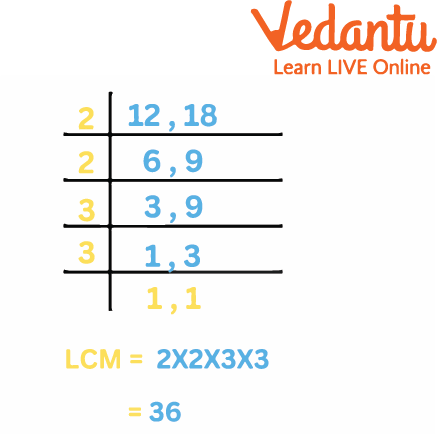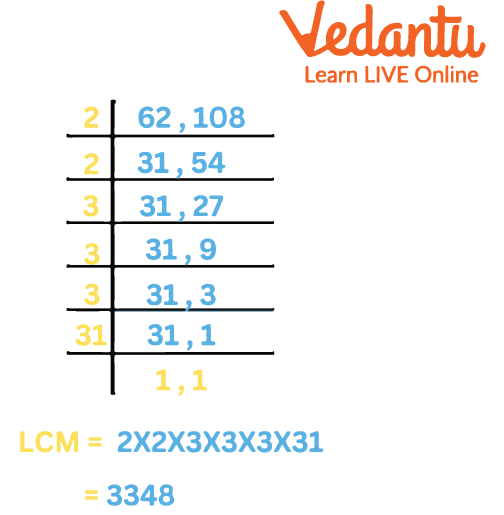Courses
Courses for Kids
Free study material
Offline Centres
More

# LCM by Long Division MethodLast updated date: 01st Dec 2023
Total views: 100.5k
Views today: 3.00k## How Do We Find Out the LCM of a Number?

LCM by division is the process of finding the least common multiple of a given group of integers by dividing them by a common prime number. For this, we need to completely understand the prime factors of the given numbers. Prime factorization and listing the multiples are two additional techniques for calculating LCM.

### LCM by Division Method

The LCM by division technique finds the LCM (Least Common Multiple) of integers by reducing them by shared prime numbers. This technique of determining the LCM of numbers is one of the standards and provides a rapid result. We must understand the common multiplication tables and the prime numbers that can totally divide the provided values.

### LCM by Common Division Method

To obtain the LCM using the common division method, we must first determine the prime factors of the provided integers.

Step 1: Take the provided numbers and divide them by the least prime integer.

Step 2: In the second row, write the quotient and the number that is not divisible by the previous prime number.

Step 3: Divide the integers by the next lowest prime number.

Step 4: Keep dividing until the remaining is a prime number or one.

Step 5: To find the LCM, multiply all the divisors and the remaining prime number (if any).

## Finding LCM by Long Division Method

To find an LCM by long division method let us take an example and understand this concept in a better way.

Find the LCM of 12 and 18 by the long division method.

Step one: The least prime number of the numbers 12 and 18 is 2. So we will divide both the numbers by 2.

Step two: Write the quotient below the number. The quotients further can be divided by 2.

Step three: The new quotient obtained is written below the previous one. As 9 wasn't divisible by 2 so it is written as it is in the next line.

Step four: Now we can divide 3 and 9 by 3, as it is the least prime number that can divide both 3 and 9.

Step five: We will repeat the step till 1 is obtained. Multiply all the divisors. The result would be LCM of 12 and 18.LCM of 12 and 18 by Long Division Method

## Sample Questions

1. Find the LCM of 62 and 108.

a. 3348

b. 3267

c. 4577

d. 3356

Ans: 3348

Explanation: To find the LCM using the long division method, divide 62 and 108 by the prime number and obtain a quotient that can be further divided by the prime number. Repeat the steps till you get 1 as the quotient. Multiply all the divisors to obtain LCM of 62 and 108.Example Of LCM

2. LCM of any prime number is

a. The number is less than it.

b. The number itself

c. 1

d. None of the above

Ans: The number itself.

Explanation: The LCM of the prime number would be the number itself as the prime numbers are not divisible by any other number than by themselves. For example, 2, 3, and 5 are not divisible by any other number than themselves.

3. We get the remainder by using the division method for finding LCM.

a. True

b. False

Ans: False

Explanation: We only get the divisor and the quotients while doing the division method for finding the LCM.

## Conclusion

LCM is the least common multiple and can be calculated using the division method. In this, the divisor is the prime number that would be divisible by the set of numbers given as the dividend. We need to divide till we obtain a prime number or 1. Then we can multiply all the divisors and find the value of LCM.

## FAQs on LCM by Long Division Method

1. What are the various ways for calculating the LCM of numbers?

The following are the several ways for determining the LCM of numbers:

• Method of Prime Factorization

• Division Method

• Method of listing multiples

2. How do you calculate the LCM of two integers using the prime factorization method?

Step 1: Write the integers' prime factorization.

Step 2: Obtain the LCM of the provided number by multiplying the prime elements raised to their maximum power.

3. How to Find the LCM of Three Numbers Using the Division Method

The LCM of three numbers may be computed using division in the same manner that it is for two numbers. We use commas to separate them and look for prime integers that can split them. The quotient is written below the integers, while the divisors are written on the left. We divide each row again and again until we have 1 as the quotient of all the numbers. The LCM of the provided integers is then calculated by multiplying the divisors written on the left by the divisors written on the right.

4. What is the difference between HCF and LCM?

In HCF we only multiply the factors which are common in both numbers but in LCM we multiply all the factors of both numbers.